Skip to main content

# Coronary stent as a tubular flow heater in magnetic resonance imaging

## Abstract

### Background

A coronary stent is an artificial metallic tube, inserted into a blocked coronary artery to keep it open. In magnetic resonance imaging (MRI), a stented person is irradiated by the radio-frequency electromagnetic pulses, which induce eddy currents in the stent that produce Joule (resistive) heating. The stent in the vessel is acting like a tubular flow heater that increases the temperature of the vessel wall and the blood that flows through it, representing a potential hazard for the stented patient.

### Methods

Heating of a metallic coronary stent in MRI was studied theoretically and experimentally. An analytical theoretical model of the stent as a tubular flow heater, based on the thermodynamic law of heat conduction, was developed. The model enables to calculate the time-dependent stent’s temperature during the MRI examination, the increase of the blood temperature passing through the stent and the distribution of the temperature in the vessel wall surrounding the stent. The model was tested experimentally by performing laboratory magnetic resonance heating experiments on a non-inserted stainless-steel coronary stent in the absence of blood flow through it. The model was then used to predict the temperature increase of the stainless-steel coronary stent embedded in a coronary artery in the presence of blood flow under realistic MRI conditions.

### Results

The increase of the stent’s temperature and the blood temperature were found minute, of the order of several tenths of a degree, because the blood flow efficiently cools the stent due to a much larger heat capacity of the blood as compared to the heat capacity of the stent. However, should the stent in the vessel become partially re-occluded due to the restenosis problem, where the blood flow through the stent is reduced, the stent’s temperature may become dangerously high.

### Conclusions

In the normal situation of a fully open (unoccluded) stent, the increase of the stent temperature and the blood temperature exiting the stent were found minute, of less than 1°C, so that the blood flow efficiently cools the stent. However, should the problem of restenosis occur, where the blood flow through the stent is reduced, there is a risk of hazardous heating.

## Background

In medicine, a stent is an artificial “tube” inserted into a natural passage/conduit in the body to prevent, or counteract, a disease-induced, localized flow constriction. The most widely known stent use is in the coronary arteries (Hubner 1998), by employing a bare-metal stent, a drug-eluting stent or occasionally a covered stent. Coronary stents are inserted during a percutaneous coronary intervention (PCI) procedure to keep the blocked coronary arteries open. Stents are also applied to the urinary tract (Yachia 1998), where ureteral stents are used to ensure patency of a ureter, which may be compromised, for example, by a kidney stone. Prostatic stents (Yachia 1998) are needed if a man is unable to urinate due to an enlarged prostate. Stents are also used in a variety of vessels aside from the coronary arteries and as a component of peripheral artery angioplasty.

A coronary stent is a tubular metal mesh, attached initially in its collapsed form onto the outside of a balloon catheter. In the angioplasty procedure, the physician threads the stent through the lesion in the vessel and expands the balloon that deforms the stent to its expandable size by matching the undeformed vessel diameter. After removal of the deflated balloon, the framework of the stent remains in direct contact with the vessel wall (Figure 1), where it is overgrown by the endothelial tissue in the course of subsequent months. The coronary stent remains permanently inserted in the vessel, representing a metallic object firmly incorporated into the human body for life.Figure 1

Stents can be assembled from a range of metallic alloys, including stainless steel, nitinol (nickel–titanium) and cobalt–chromium. The presence of a metallic object in the body brings up several safety issues in Magnetic Resonance Imaging (MRI) diagnostics. The two most important are the influence of magnetic forces on the implanted stent (Ahmed and Shellock 2001; Jost and Kumar 1998; Scott and Pettigrew 1994; Shellock and Shellock 1999; Strohm et al. 1999; Kagetsu and Litt 1991; Woods 2007; Lopič et al. 2013) and the stent heating by the radio-frequency (rf) electromagnetic pulses (Shellock and Morisoli 1994; Nyenhuis et al. 2005; Shellock 2011). In MRI, a stented person is irradiated by the rf pulses, which induce eddy currents in the stent that produce Joule (resistive) heating of the stent and its surroundings. During the MRI, the stent in the vessel is acting much like a tubular flow heater that increases the temperature of the vessel wall and the blood that flows through it. Due to the possible heat-induced protein coagulation and the formation of blood clots, stent heating in MRI deserves careful attention. In this paper, we present an analytical theoretical model of the stent as a tubular flow heater in the MRI examination. The model is based on the thermodynamic law of heat conduction and enables us to calculate the time-dependent stent temperature during the MRI examination, the increase of the blood temperature passing through the stent and the distribution of the temperature in the vessel wall surrounding the stent. We have tested the model experimentally by performing laboratory magnetic resonance rf heating experiments on a non-inserted stainless-steel coronary stent in the absence of blood flow through it and good matching to the theory was found. The model was then used to predict the temperature increase of the stainless-steel coronary stent embedded in a coronary artery in the presence of blood flow through it under realistic MRI conditions. The results indicate that the increase of the stent’s temperature and the blood temperature are minute, of the order of several tenths of a degree, because the blood flow efficiently cools the stent due to a much larger heat capacity of the blood as compared to the heat capacity of the stent. However, should the problem of restenosis occur, where the stent in the vessel becomes partially re-occluded and the flow reduces, there is a risk of hazardous heating and the stent’s temperature may become dangerously high.

## Methods

### Stent description and characterization

Our experiments were performed on a commercial balloon-expandable coronary stent (HORUSS HDS 1625, International Biomedical Systems, Trieste). The stent was fabricated of a surgical stainless steel, having the nominal length of 16 mm, the nominal diameter of 2.5 mm and the nominal pressure for the expansion of 8 atm. A small part of the stent was cut away for the physical property determination of the material, whereas the large part (12 mm length) was used for the rf heating experiments. The photograph of the employed stent is shown in Figure 2a.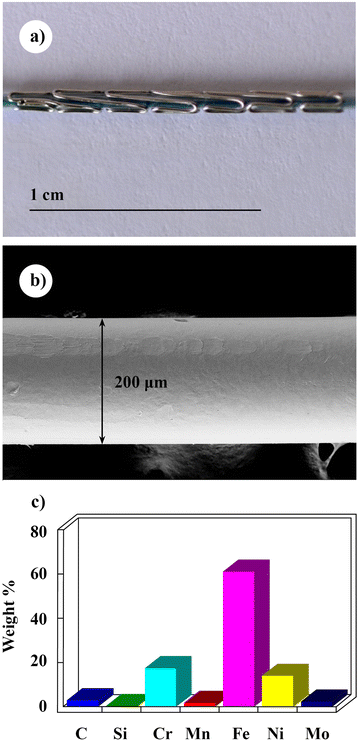Figure 2

The stent’s material was surgical stainless steel type 316LVM (where “L” stands for Low Carbon and “VM” denotes Vacuum Melted), using a wire of 200–μm diameter (Figure 2b). The 316LVM is an austenitic steel, which is widely used for implants because it never develops surface rust and shows superior resistance to constant salt water exposure. According to the international standards (ASM International Handbook Committee 1990), the elemental composition of 316LVM is in the range 0.03 wt.% C, up to 2.0% Mn, 2.0 – 3.0% Mo, up to 1.0% Si, 16.0 – 18.0% Cr, 10.0 – 14.0% Ni, up to max. 0.16% N, and about 65% Fe as the majority element. We have determined the particular chemical composition of our investigated stent by the energy-dispersive X-ray spectrometer (EDS) using a scanning electron microscope (SEM) Supra VP 35 Zeiss (Carl Zeiss AG, Oberkochen, Germany). The resulting chemical composition (in weight %) is displayed as a histogram in Figure 2c. The too high carbon concentration (2.94%) is artificial; it originates from the contamination of the SEM by the hydrocarbons (a known problem in SEM microscopy). Apart from the carbon, other elements are within the specifications for the 316LVM steel.

Since the induction of the eddy currents by the rf field is strong in metallic alloys of low electrical resistivity, we have measured the electrical resistivity ρ of the stent’s wire. The direct current (dc) resistivity was determined in the temperature interval from 2 to 360 K by a standard four-contact method using a Quantum Design PPMS (Physical Property Measurement System). The dc resistivity is usually good approximation to the frequency-dependent (ac) resistivity ρ(ω) up to the microwave frequencies, so that it is considered valid also in the radio-frequency range of the MRI experiments. The result is shown in Figure 3, where it is evident that ρ(T) exhibits a positive temperature coefficient at the values in the range ρ ≈ 100 – 150μΩcm, typical of moderately electrically conducting alloys. At the body temperature of 37°C, the resistivity amounts $${\rho}_{37^oC}=\kern0.75em 146\upmu \Omega \mathrm{cm},$$ which is low enough that a significant induction-heating effect may be expected under the rf-pulse irradiation.Figure 3

To verify the analytical model of the stent as a tubular flow heater presented in this paper, the specific heat c s of the stent must be known. We have determined c s experimentally by using a Quantum Design PPMS. The graph of the temperature-dependent c s in the interval from 2 to 370 K is presented in Figure 4, showing that at 37°C, c s  = 0.46 J/gK.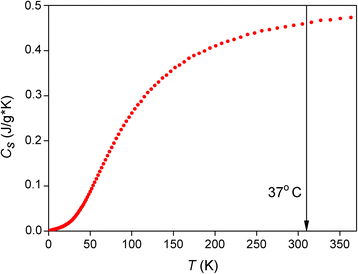Figure 4

### Rf heating experiments

Rf heating experiments of the stent were conducted in a standard 4.7 T vertical-bore NMR spectrometer operating at the proton resonance frequency ν 0 (1H) = 200 MHz. An AMT 300 W rf power transmitter was used. To monitor the temperature of the stent, a platinum Pt100 resistor was glued to its outer surface by a thermally conducting varnish (GE/IMI 7031). The stent with the sensor was wrapped up into a Teflon foil of 1.0 mm total thickness. The output of the sensor was connected to a data logger (PT-104 PT 100 Converter, Pico Technology, Cambridgeshire, UK) that has digitized the signal and enabled us to follow the time-dependent increase of the stent’s temperature under the rf irradiation.

## Results and discussion

### Analytical model of the stent as a tubular flow heater in MRI

We approximate the actual stent’s geometry of a cylindrical metal mesh by a homogeneous thin cylinder of the length l and the radius R (Figure 5). The mass of the stent is m s and its specific heat at constant pressure is c s . The stent is surrounded by the vessel wall of cylindrical shape with the wall thickness d and the thermal conductivity λ. The stent’s temperature is T s , whereas the temperature at the outer surface of the vessel is T 0 (taken roughly as the body temperature 37°C). The blood mass flow Φ b  = dm b /dt (where m b is the mass of the blood) enters the stent at the body temperature T 0 and exits at an elevated temperature T b . Due to the heart pulsing, the blood flow is pulsed, but we approximate it by a stationary flow $${\varPhi}_b={\rho}_b{S}_s{\overline{v}}_b$$, where ρ b is the blood density, S s  = π R 2 is the stent’s cross section and $${\overline{v}}_b$$ is the average blood velocity through the stent. The specific heat at constant pressure of the blood is c b .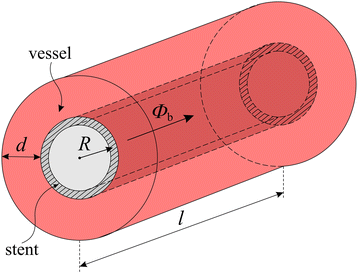Figure 5

The rf electromagnetic pulses during the MRI examination supply the stent with the rf power P rf , which transforms into the heat dQ = P rf dt via the Joule heating by the induced eddy currents. A part of this heat is released through the vessel wall. For a cylindrical vessel, the thermal power (heat flow) P v through the wall amounts (Halliday et al. 2005)

$${P}_v=\frac{\lambda 2\pi l}{ \ln \left(1+d/R\right)}\left({T}_s-{T}_0\right).$$
(1)

The blood flow through the stent takes away the thermal power

$${P}_b={\varPhi}_b{c}_b\left({T}_b-{T}_0\right).$$
(2)

The heat imbalance (P rf  − P v  − P b ) dt increases the stent’s temperature by dT s ,

$$\left({P}_{rf}-{P}_v-{P}_b\right)\;dt={m}_s{c}_sd{T}_s.$$
(3)

Using Equations 1 and 2, we rewrite Equation 3 in the form

$${P}_{rf}-\frac{\lambda 2\pi l}{ \ln \left(1+d/R\right)}\left({T}_s-{T}_0\right)-{\varPhi}_b{c}_b\left({T}_b-{T}_0\right)={m}_s{c}_s\frac{d{T}_s}{dt},$$
(4)

which contains two unknown variables, T s (t) and T b (t). To proceed, we assume that the time-dependence of the blood temperature T b follows the time-dependence of the stent’s temperature T s , though T b may be lower than T s ,

$${T}_b-{T}_0=\varepsilon \left({T}_s-{T}_0\right),$$
(5)

with 0 ≤ ε ≤ 1. Equation 4 is then cast into the form

$$\frac{d{T}_s}{dt}+\alpha \left({T}_s-{T}_0\right)=\frac{P_{rf}}{m_s{c}_s},$$
(6)

where

$$\alpha =\frac{1}{m_s{c}_s}\left(\frac{\lambda 2\pi l}{ \ln \left(1+d/R\right)}+\varepsilon\;{\varPhi}_b{c}_b\right).$$
(7)

The solution of Equation 6 is

$${T}_s={T}_0+\varDelta T\left(1-{e}^{-\alpha\;t}\right),$$
(8)

where ΔT = P rf /α m s c s . The stent reaches the new steady-state temperature T s (t → ∞) = T 0 + ΔT exponentially with the time constant α − 1 after the start of the rf pulsing in the MRI examination. Using the definition of α, the increase of the stent’s temperature can also be written in the form

$$\varDelta T=\frac{P_{rf}}{\frac{\lambda 2\pi l}{ \ln \left(1+d/R\right)}+\varepsilon {\varPhi}_b{c}_b}.$$
(9)

The time-dependent stent’s temperature given by Equation 8 is shown in Figure 6a.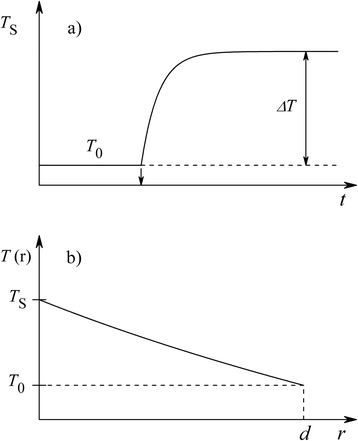Figure 6

According to the above model, the blood temperature T b (t) follows the stent’s temperature T s (t), as expressed by Equation 5. The efficiency of the heat transfer from the stent to the blood is given by the empirical factor ε, which assumes a value between 1 and 0, depending on the details of the vessel and the blood flow through it. ε should be determined experimentally for a particular vessel and the type of inserted stent. The time-dependent blood temperature obeys the equation T b (t) = T 0 + ε (T s (t) − T 0), where T s (t) is given by Equation 8. The T s (t) curve shown in Figure 6a, scaled by a factor ε, is thus valid for the blood temperature T b (t) as well. After the steady-state is reached during the irradiation, the increase of the blood temperature passing the stent is εΔT with ΔT given by Equation 9.

The radial distribution of the temperature T(r) within the vessel wall surrounding the stent (0 ≤ r ≤ d) in the steady state is obtained by assuming a stationary radial heat flow through the wall, so that P v as given by Equation 1 is constant at any radial distance r from the stent. The condition

$${P}_v=\frac{\lambda 2\pi l\left({T}_s-T(r)\right)}{ \ln \left(1+r/R\right)} = const.$$
(10)

yields the T(r) dependence

$$T(r)={T}_s-\frac{P_v \ln \left(1+r/R\right)}{\lambda 2\pi l},$$
(11)

which is shown in Figure 6b. At the contact surface to the stent, the vessel wall heats up to the stent temperature, T(r = 0) = T s , whereas at the vessel’s outer surface, the temperature drops to the body temperature, T(r = d) = T 0, via a ln(1 + r/R) radial dependence.

In the above analytical model of the stent as a tubular flow heater, some simplifications were used mostly to ensure mathematical tractability of the calculation. Two of them deserve to be discussed in more detail. In the model, the vessel wall surrounding the stent is considered to be cylindrical of the thickness d, where the temperature at the contact surface to the stent is assumed to equal the stent temperature T s, whereas at the outer surface it equals the body temperature T 0. The vessel wall thickness was not specified, but for the coronary arteries and the coronary stents it is reasonable to assume the inequality d < R, where R is the radius of the stent. This approximation assumes a very powerful cooling process in the body and an excessive temperature gradient within the vessel wall. In fact human temperature regulation is not powerful enough to justify this assumption. In reality, the temperature in the tissue surrounding the stent will drop to the body temperature over the distance of the order of several R, thus considerably larger than the vessel wall thickness. In the case where the vessel and the tissue behind it have similar thermal conductivity λ values (a reasonable assumption), this discrepancy can be removed by simply taking larger d value in Equations 1 to 9. Since d always appears in a logarithmic function of the form ln(1 + d/R), which is located in the denominator of the expression for ΔT (Equation 9), larger d will reduce the heat flow P v through the vessel wall. Due to the logarithmic dependence on d, the changes are relatively weak (e.g., for a thickness increase from d = R to d = 10R, the function ln(1 + d/R) increases by a factor 3.4 only). Smaller P v will increase the stent’s steady-state temperature ΔT, thus subjecting the inner side of the vessel to higher temperatures and also heating stronger the blood flowing through the stent. The heating effect is thus increased under the assumption that the temperature of the tissue surrounding the stent drops to the “unperturbed” body temperature at a distance considerably larger than the stent’s diameter R.

The second simplification is the assumption that the blood temperature follows the stent temperature, as expressed by Equation 5. This assumption has enabled the elimination of one unknown variable (the blood temperature T b (t)) from the calculation and kept the model simple and analytically tractable. The assumption required the introduction of the empirical “heat-transfer efficiency factor” ε (with 0 ≤ ε ≤ 1,) describing the efficiency of the heat transfer from the stent to the blood. While it is intuitively plausible to relate the blood temperature to the stent temperature by the Equation 5, the factor ε is not well-defined as the stent-to-blood heat transfer efficiency will depend on the details of the vessel, the type and geometry of the inserted stent and the blood flow through it. Weaker heat transfer (ε → 0) will increase the risk of high temperatures developed in the stent, as the stent is not giving up the heat to the blood. Good heat transfer can generally be expected for longer stents and lower blood velocity.

### Rf heating experiments on a coronary stent in the absence of blood flow

In our experimental study of the temperature increase of the stainless-steel coronary stent subjected to irradiation by the rf pulses, the stent with the attached Pt100 thermometer was placed into the rf coil of the NMR probe head and inserted into the magnet. A typical MRI rf pulse sequence contains one or more pulses that are usually shaped in the time domain, where a truncated sinc and a Gaussian shape are most commonly employed (Callaghan 1991). Instead of using shaped pulses, our pulse sequence was composed of a train of 50 rectangular pulses of τ = 20 μs length each (1 ms total pulse duration), separated by 5 μs and repeated with the repetition rate t 0 = 100 ms (Figure 7), yielding the duty cycle δ D  = 0.01. A shaped rf pulse in a realistic MRI experiment has a similar duration and repetition rate. The power of the rf pulses has been varied during the experiment and the temperature raise of the stent was monitored at different power levels. Here it is worth mentioning that the actual shape of the rf pulses is relatively unimportant for the heating effect; what matters is the average rf power delivered by the pulses to the stent.Figure 7

The irradiation of the stent started by switching on the pulse sequence of Figure 7 at a given moment of time and then continuously repeating it at a selected transmitter rf output power level. This resulted in rapid initial growth of the stent temperature, which has saturated to a constant plateau after some time when the balance between the incoming rf energy and the outgoing heat due to the thermal conduction was achieved. In order to minimize heat losses by thermal conduction to the surrounding air, the rf probe head with the stent was placed into an Oxford continuous-flow cryostat CF 1200 (Oxford Instruments, Abingdon, Oxfordshire, UK), where the air could be evacuated to a pressure down to 0.2 bar. After a steady-state temperature was reached with time at a given rf power level, the transmitter power was increased and the new stent’s temperature was recorded. In the following we present the stent’s temperature increase as a function of the average rf power over the pulse sequence repetition time t 0, defined as $$\overline{P}=\left(1/{t}_0\right){\int}_0^{t_0}P(t)\kern0.5em dt}={\delta}_D{P}_{tr},$$ where P tr is the transmitter power (e.g., $$\overline{P}\kern0.5em = 3\ \mathrm{W}$$ for the full transmitter power P tr  = 300 W and the duty cycle δ D  = 0.01). The time-dependent stent’s temperature under the rf irradiation at different average power levels $$\overline{P}\kern0.5em = 0.1,\ 0.3,\ 1,\ \mathrm{and}\ 3\ \mathrm{W}$$ is shown in Figure 8a. The initial stent’s temperature was 19.5°C. We observe that at each power level, the rapid initial increase of the temperature slows down with time and reaches a steady state in about 100 s. For the highest average rf power of $$\overline{P}\kern0.5em = 3\ \mathrm{W},$$ the stent has reached an astonishingly high temperature of 52.5°C (yielding an increase by as much as ΔT = 33°C from the initial temperature before the irradiation). The values of the steady-state temperature increase ΔT, as a function of $$\overline{P}$$, are given in Table 1. The detailed shape of the stent’s time-dependent temperature T(t) under irradiation by the rf pulses for the initial power level of $$\overline{P} = 0.1\ \mathrm{W}$$ (enclosed in a dashed box in Figure 8a), is shown expanded in Figure 8b, where an exponential increase is obvious. We have also checked the increase of the stent’s temperature in the air environment at the ambient pressure of 1 bar, where the stent’s heat supplied by the rf irradiation is taken away more efficiently by the thermal convection to the surrounding air. The highest temperature of the stent in the 1 bar air atmosphere for $$\overline{P}\kern0.5em = 3\ \mathrm{W}$$ has dropped to 48°C, as compared to 52.5°C in an identical experiment in the reduced 0.2 bar atmosphere, showing that the thermal convection to the air takes away considerable amount of the heat from the stent.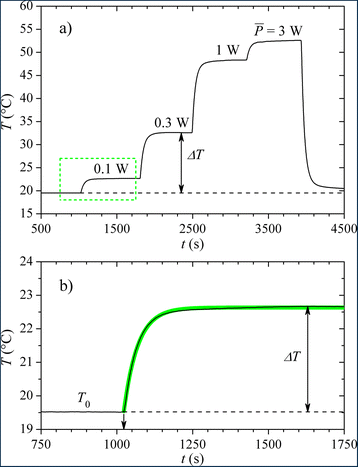Figure 8

The above results indicate the possibility of hazardous heating of the stent in the MRI examination by as much as ΔT ≈ 30°C. It is, however, important to emphasize that this conclusion is based on experiments with no blood flow through the stent. We shall discuss in the following how does this conclusion change under the blood flow condition.

### Comparison between the theory and experiment

The stent’s temperature T s (t) in our rf heating experiment of Figure 8b was reproduced theoretically by the Equation 8. Excellent fit (thick green curve in Figure 8b) was obtained using the parameters ΔT = 3.1°C and the time constant α − 1 = 45 s, confirming that T s reaches the new steady-state value exponentially in time. The experimental ΔT and α − 1 values were compared to the theoretical values, calculated from Equations 9 and 7. In the absence of the blood flow, ΔT = P rf /[λ2π l/ln(1 + d/R)]. The role of the vessel wall in the experiment was played by the Teflon jacket of the thickness d = 1 mm and the thermal conductivity (at 25°C) λ = 0.25 W/mK around the stent. Taking the employed $${P}_{rf}=\overline{P}=0.1\ \mathrm{W},$$ and using the stent’s geometrical parameters l = 12 mm and R = 1.25 mm, we obtain ΔT = 3.1°C, a value that matches perfectly the experimental one. The parameter α = [λ2π l/ln(1 + d/R)]/m s c s was calculated by using the stent mass m s  = 21.7 mg and the specific heat value at 37°C c s  = 0.46 J/gK, yielding α − 1 = 0.3 s. The theoretical time constant α − 1 is a factor 150 smaller than the experimental one, so that the theory predicts a much faster increase of the stent temperature than observed experimentally. This discrepancy can be understood by noticing that in our experiment, a Pt100 sensor was rigidly attached to the stent and was heated up together with it. The thermal conductivity of the Pt100 ceramic housing is much lower than that of the metallic stent, so that the combined stent–Pt100 system has reached the new steady state temperature in a considerably longer time that it would be reached by the metallic stent alone.

### Application of the model to the coronary stent in the presence of blood flow

In order to predict the temperature increase of the stainless-steel coronary stent embedded in a coronary artery in vivo in a realistic MRI examination, we calculate the parameter ΔT for our investigated stent in the presence of the blood flow. We take the geometrical parameters of the as-fabricated stent l = 16 mm and R = 1.25 mm. For the coronary artery, we take the following order-of-magnitude estimated parameters: the wall thickness d = 1 mm and the thermal conductivity λ = 1 W/mK (this value was estimated from the reported thermal conductivity of the human skin plus fat that amounts to 0.73 W/mK at 36°C and the thermal conductivity of muscles that amounts to 1.91 W/mK at 36°C (Ducharme and Tikuisis 1991)). For the blood flow through the stent we take a typical volume flow through a coronary artery Φ bV  = 2 ml/s (Spaan 1991). Since the blood density is ρ b  = 1.06 g/cm3, this yields the blood mass flow Φ b  = ρ b Φ bV  = 2 g/s. 2 g/s. The specific heat of the blood is c b  = 3.78 J/gK. For the stent-to-blood heat transfer efficiency parameter we take an ad hoc value ε = 0.5. P rf is taken arbitrary as 3 W (recall that at this rf power, the stent has heated up by as much as ΔT = 33°C in the absence of the blood flow, as shown in Figure 8a).

Using the above parameter values, the increase of the stent’s temperature was calculated from Equation 9 to amount ΔT = 0.8°C only, whereas the blood temperature increase is by εΔT =  0.4°C. This ΔT value is minute as compared to the case where there is no blood flow through the stent. In the absence of the blood flow (setting Φ b  = 0 in Equation 9), the increase of the stent’s temperature would be considerable, ΔT = 17.6°C. The reason for the smallness of the ΔT value in the presence of the blood flow becomes evident by inspecting the denominator of Equation 9 that contains two terms. The first term λ2π l/ln(1 + d/R) = 0.17 W/K originates from the heat flow through the vessel wall, whereas the second term εΦ b c b  = 3.78 W/K originates from the heat taken away by the blood flow. The second term is much larger than the first one, εΦ b c b /[λ2π l/ln(1 + d/R)]  = 22, so that the blood flow efficiently cools the stent in the MRI examination, owing to the much larger heat capacity of the blood, C b  = m b c b , as compared to the heat capacity of the stent, C s  = m s c s (where the ratio of the specific heats is c b /c s  = 8.2). The estimated increase of the stainless-steel coronary stent temperature by ΔT = 0.8°C during MRI in vivo is thus small enough to be considered harmless to the human body.

### The problem of restenosis

The above result of a minute increase of the stent’s temperature due to the efficient cooling by the blood flow applies to the normal situation, where the stent’s cross section is large enough to enable the rated (unrestricted) blood flow through a coronary vessel, i.e., the stent is fully “open”. However, the problem of restenosis is sometimes encountered after the stent insertion in the course of months or years, where the stent becomes partially re-occluded and the flow reduces. Consequently, the reduced flow is no more capable of efficiently cooling the stent in the MRI examination of a patient with a partially re-occluded stent and there may appear a risk of hazardous heating of the stent’s surroundings. Defining the blood flow reduction factor $$x=\left(1-{\varPhi}_b/{\varPhi}_b^0\right)\times 100$$ (in %) with $${\varPhi}_b^0$$ denoting the unrestricted flow, we are able to predict from Equation 9 the temperature increase of the stent ΔT for an arbitrary reduced blood flow. The x = 0% value corresponds to the fully open stent (no flow reduction), whereas x = 100% corresponds to the fully blocked stent (100% flow reduction). For the calculation we took the same stent and vessel parameters as before (recall that for these parameters, the model yielded ΔT = 0.8°C for the fully open stent, whereas ΔT = 17.6°C for the fully blocked stent). The graph of the stent’s temperature in the body, T s  = 37°C + ΔT, as a function of the blood flow reduction, is shown in Figure 9. We observe that for the flow reduction between 0 and 90%, the stent’s temperature increase is relatively small (T s increases from 37.8°C at 0% reduction to 42.5°C at 90%), whereas T s increases drastically for the high flow reduction between 90% and 100% (from 42.5°C at 90% to 54.6°C at 100% reduction). This behavior is again a consequence of the much larger heat capacity of the blood as compared to the stent, demonstrating that even a substantially diminished blood flow through the stent is still able to cool it efficiently. In contrast, dangerously high temperatures are developed in the body for the stent occlusion close to 100%.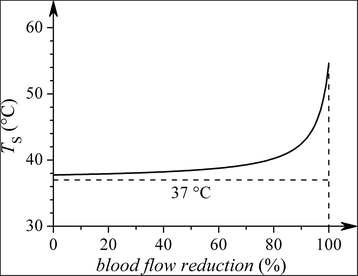Figure 9

In the MRI examination, the patient is irradiated by the rf pulses typically for a time of about 20 – 30 minutes, which represents the time during which the stent “heater” is switched on. The long heater switch-on time and the high enough temperature for the heat-induced protein coagulation and the formation of blood clots represent a risk of hazardous heating in the stent’s surroundings during the MRI of a stented patient with a high stent occlusion between 90% and 100%. Restenosis thus represents a potentially hazardous heating problem in MRI.

## Conclusions

We have investigated the heating of a metallic coronary stent in a MRI examination. The experimental results presented in this paper are valid for the particular geometrical parameters (length and diameter) of the employed stent, the 316LVM stainless steel material, the proton resonance frequency of 200 MHz (corresponding to the 4.7 T magnetic field) and the average rf irradiation power up to 3 W. For the highest average rf power of 3 W employed in our experiments on the stent in the absence of the blood flow through it, the stent has heated up by as much as ΔT = 33°C from the initial temperature before the irradiation. The experimental and theoretical methodologies applied in this study are, however, suitable to investigate other types of stents with different geometries, fabricated of different metallic alloys, e.g., nickel–titanium and cobalt–chromium and other rf irradiation strengths.

The metallic coronary stent acting as a tubular flow heater in the MRI examination was also modeled theoretically, by considering that the stent receives the energy from the rf electromagnetic field and heats up the surrounding vessel and the blood flowing through it. The analytical model has successfully reproduced the exponential increase of the stent’s temperature during our rf heating experiments in the absence of blood flow through the stent. The model was then used to predict the increase of the temperature of the stainless-steel coronary stent embedded in a coronary artery in the presence of blood flow through it, mimicking an in vivo realistic MRI examination. In the normal situation of a fully open (unoccluded) stent, the increase of the stent’s temperature as well as the increase of the blood temperature exiting the stent were found minute, of less than 1°C, so that the blood flow efficiently cools the stent. This is a consequence of the much larger heat capacity of the blood as compared to the heat capacity of the stent. However, should the problem of restenosis occur with time after the stent insertion, where the stent in the vessel becomes partially re-occluded and the flow reduces, there is a risk of hazardous heating. The temperature of the occluded stent may become dangerously high to enable protein coagulation and the formation of blood clots in the stent’s surroundings.

## References

1. Ahmed S, Shellock FG (2001) Magnetic resonance imaging safety: implications for cardiovascular patients. J Cardiovasc Magn Reson 3:171–182

2. ASM International Handbook Committee (1990) Metals handbook, vol 1, 10th edn. ASM International Handbook Committee, Ohio Park

3. Callaghan PT (1991) Principles of nuclear magnetic resonance microscopy. Clarendon Press, Oxford, p 100

4. Ducharme MB, Tikuisis P (1991) In vivo thermal conductivity of the human forearm tissues. J Appl Physiol 70:2682–2690

5. Halliday D, Resnick R, Walker J (2005) Fundamentals of Physics, 7th edn. John Wiley & Sons, New York, p 493

6. Hubner PJB (1998) Guide to coronary angioplasty and stenting. Harwood Academic Publishers, Amsterdam

7. Jost C, Kumar V (1998) Are current cardiovascular stents MRI safe? J Invas Cardiol 10:477–479

8. Kagetsu ND, Litt AW (1991) Important considerations in measurement of attractive forces on metallic implants in MR imaging. Radiology 179:505–508

9. Lopič N, Jelen A, Vrtnik S, Jagličić Z, Wencka M, Starc R, Blinc A, Dolinšek J (2013) Quantitative determination of magnetic force on a coronary stent in MRI. J Magn Reson Imaging 37:391–397

10. Nyenhuis JA, Park SM, Kamondetdacha R, Amjad A, Shellock FG, Rezai A (2005) MRI and implanted medical devices: basic interactions withan emphasis on heating. IEEE Trans Device Mat Rel 5:467–478

11. Scott NA, Pettigrew RI (1994) Absence of movement of coronary stents after placement in a magnetic resonance imaging field. Am J Cardiol 73:900–901

12. Shellock FG (2011) Reference manual for magnetic resonance safety, implants and devices. Biomedical Research Publishing Group, Los Angeles, pp 246–251

13. Shellock FG, Morisoli SM (1994) Ex vivo evaluation of ferromagnetism, heating, and artifacts for heart valve prostheses exposed to a 1.5 Tesla MR system. J Magn Reson Imaging 4:756–758

14. Shellock FG, Shellock VJ (1999) Metallic stents: evaluation of MR imaging safety. Am J Roentgenol 173:543–547

15. Spaan JAE (1991) Coronary blood flow: mechanics, distribution and control. Kluwer Academic Publishers, Dordrecht

16. Strohm O, Kivelitz D, Gross W, Schulz-Menger J, Liu X, Hamm B, Dietz D, Friedrich MG (1999) Safety of implantable coronary stents during 1H-magnetic resonance imaging at 1.0 and 1.5 T. J Cardiovasc Magn Reson 1:239–245

17. Woods TO (2007) Standards for medical devices in MRI: present and future. J Magn Reson Imaging 26:1186–1189

18. Yachia D (1998) Stenting the urinary system. Isis Medical Media, Oxford

Download references

## Author information

Authors

### Corresponding author

Correspondence to Janez Dolinšek.

## Additional information

### Competing interests

The authors declare that they have no competing interests.

### Authors’ contributions

SV carried out the stent heating experiments. MW carried out the physical-property measurements of the stent’s material. AJ carried out the scanning electron microscopy experiments and the EDS compositional analysis. HJK participated in the application of the theoretical model to the experimental data. JD conceived of the study, developed the analytical model of a stent as a tubular flow heater, and performed the coordination. All authors read and approved the final manuscript.

## Rights and permissions

Open Access  This article is licensed under a Creative Commons Attribution 4.0 International License, which permits use, sharing, adaptation, distribution and reproduction in any medium or format, as long as you give appropriate credit to the original author(s) and the source, provide a link to the Creative Commons licence, and indicate if changes were made.

The images or other third party material in this article are included in the article’s Creative Commons licence, unless indicated otherwise in a credit line to the material. If material is not included in the article’s Creative Commons licence and your intended use is not permitted by statutory regulation or exceeds the permitted use, you will need to obtain permission directly from the copyright holder.

To view a copy of this licence, visit https://creativecommons.org/licenses/by/4.0/.

Reprints and Permissions